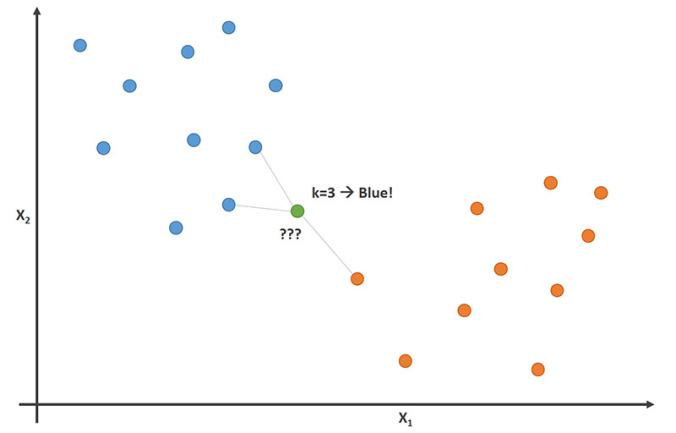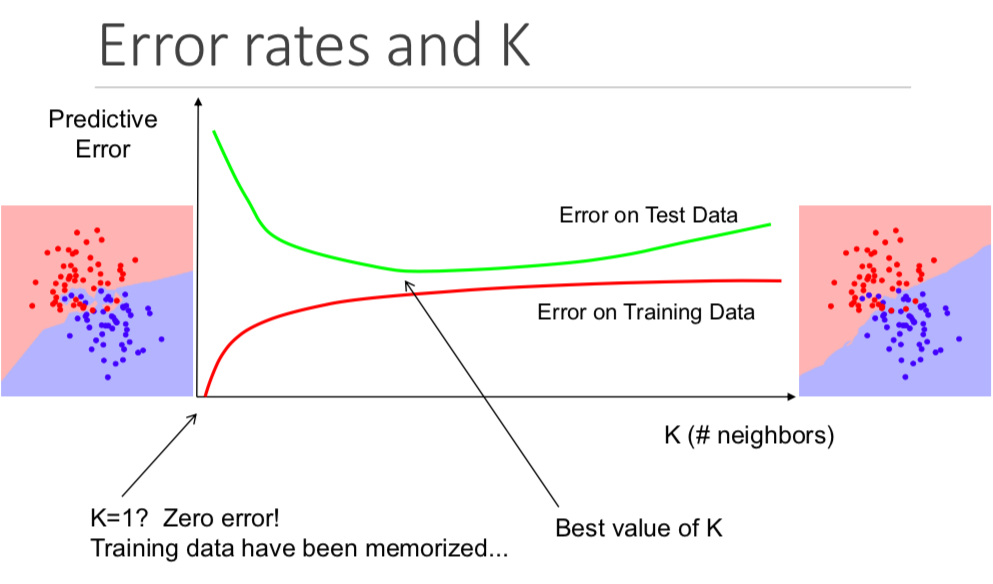# Introduction to K Nearest Neighbors Models

Hey - Nick here! This page is a free excerpt from my new eBook Pragmatic Machine Learning, which teaches you real-world machine learning techniques by guiding you through 9 projects.

In this tutorial, you will be introduced to a type of machine learning model called the K nearst neighbors algorithm.

You'll learn the theoretical underpinnings of K nearest neighbors in this tutorial before learning how to build k nearest neighbors algorithms in Python later in this course.

## What is the K Nearest Neighbors Algorithm?

The K nearest neighbors algorithm is a classification algorithm that is based on a very simple principle. In fact, the principle is so simple that it is best understood through example!

Imagine that you had data on the height and weight of football players and basketball players. The K nearest neighbors algorithm can be used to predict whether a new athlete is either a football player or a basketball player.

To do this, the K nearest neighbors algorithm identifies the K data points that are closest to the new observation.

The following image visualizes this, with a K value of `3`:In this image, the football players are labeled as blue data points and the basketball players are labeled as orange dots. The data point that we are attempting to classify is labeled as green.

Since the majority (2 out of 3) of the closets data points to the new data pints are blue football players, then the K nearest neighbors algorithm will predict that the new data point is also a football player.

## The Steps for Building a K Nearest Neighbors Algorithm

The general steps for building a K nearest neighbors algorithm are:

1. Store all of the data
2. Calculate the Euclidean distance from the new data point `x` to all the other points in the data set
3. Sort the points in the data set in order of increasing distance from `x`
4. Predict using the same category as the majority of the `K` closest data points to `x`

## The Importance of `K` in a K Nearest Neighbors Algorithm

Although it might not be obvious from the start, changing the value of `K` in a K nearest neighbors algorithm will change which category a new point is assigned to.

More specifically, having a very low `K` value will cause your model to perfectly predict your training data and poorly predict your test data. Similarly, having too high of a `K` value will make your model unnecessarily complex.

The following visualization does an excellent job of illustrating this:## The Pros and Cons of the K Nearest Neighbors Algorithm

To conclude this introductory article on the K nearest neighbors algorithm, I wanted to briefly discuss some pros and cons of using this model.

Here are some main advantages to the K nearest neighbors algorithm:

• The algorithm is simple and easy to understand
• It is trivial to train the model on new training data
• Works with any number of categories in a classification problem
• It is easy to add more data to the data set
• The model accepts only two parameters: `K` and the distance metric you'd like to use (usually Euclidean distance)

Similarly, here are a few of the algorithm's main disadvantages:

• There is a high computational cost to making predictions, since you need to sort the entire data set
• It does not work well with categorical features

## Final Thoughts

In this tutorial, you were exposed to the K nearest neighbors algorithm for the first time.

Here is a brief summary of what we learned. In the next lesson of this course, you'll code your first K nearest neighbors algorithm from scratch:

• An example of a classification problem (football players vs. basketball players) that the K nearest neighbors algorithm could solve
• How the K nearest neighbors uses the Euclidean distance of the neighboring data points to predict which category a new data point belongs to
• Why the value of `K` matters for making predictions
• The pros and cons of the `K` nearest neighbors algorithm